# Basic Components Used in Electronics & Electrical

In any electronic circuit, we come across two types of electronic component: One which response to the flow of electrical energy and either store or dissipate energy. These are the Passive Components. They can be linear components with a linear response to the electrical energy or nonlinear components with a nonlinear response to the electrical energy.

One which supplies energy or controls the flow of energy. These are the Active components. They require an external power source to be triggered and are generally used to amplify an electrical signal. Let us see every component in detail.

### 3 Passive Linear Components:

Resistor: A resistor is an electronic component that is used to resist the flow of current and cause a reduction in potential. It consists of a low conductive component joined by conducting wires at both ends. When current flows through the resistor, the electrical energy is absorbed by the resistor and dissipated in the form of heat. The resistor thus offers a resistance or opposition to the flow of current. The resistance is given as

R = V/I, where V is the voltage drop across the resistance and I is the current flowing through the resistor. The power dissipated is given by:

P = VI.

Laws of Resistance:

The Resistance ‘R’ offered by a material depends on various factors

1. Varies directly on its length, l
2. Varies inversely on its cross-section area, A
3. Depends on the nature of the material specified by its Resistivity or Specific Resistance, ρ
4. Also depends on the temperature
5. Assuming that the temperature is constant, the Resistance (R) can be expressed as R = ρl / A, Where R is resistance in ohms ( Ω ), l  is the length in meters, A is an area in square meters and ρ  is Specific Resistance in Ω-mts

A resistor’s value is calculated in terms of its resistance. Resistance is the opposition to the flow of current.

Two methods to measure resistance values:

• Using color code: Each resistor consists of a 4 or 5 color band on its surface. The first three (two) colors represent the resistor value, whereas the 4th (third) color represents the multiplier value and the last one represents the tolerance.
• Using Multimeter: A simple way to measure resistance is by using a Multimeter to measure the resistance value in ohms.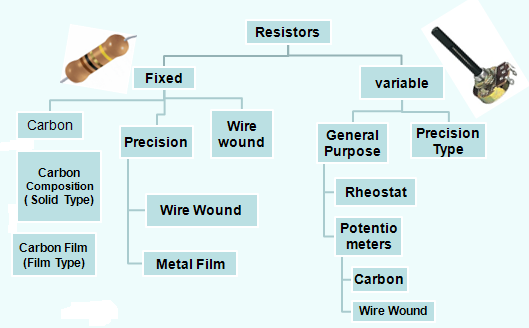### 2 Types of Resistors:

• Fixed Resistors: Resistors whose resistance value is fixed and are used to provide an opposition to the flow of current.
• They can be carbon composition resistors which are made up of a mixture of carbon and ceramic.
• They can be carbon film resistors which consist of carbon film deposited on an insulating substrate.
• They can be metal film resistor which consists of small ceramic rod coated with metal or metal oxide, with the resistance value being controlled by the thickness of the coating.
• They can be a wire-wound resistor which consists of an alloy wrapped around a ceramic rod and insulated.
• They can be surface mount resistors which consists of resistive material like tin oxide deposited on a ceramic chip.

• Variable Resistors: They provide a variation in their resistance value. They are generally used in voltage division. They can be potentiometers or presets. The resistance can be varied by controlling the wiper movement. The variable resistor or variable resistance, which consists three connections. Generally used as an adjustable voltage divider. It is a resistor with a movable element positioned by a manual knob or lever. The movable element is also called as wiper; it creates a contact with a resistive strip at any point which is selected by the manual control.

The potentiometer divides the voltage into different proportions depending on its movable positions. It is used in different circuits where we require less voltage than the source voltage.

### Practical Application of Variable Resistors:

Sometimes it is necessary to design a variable dc bias circuit that should be able to very precisely get some specific voltage to say 1.5 volts. Thus a potential divider with a variable resistor is so chosen that one can vary the voltage from 1 volt to 2 volts from a 12 volt DC battery. Not from 0 to 2 volt but 1 to 2 volt for a specific reason One can use a 10k pot across a 12-volt dc and can get that voltage but it becomes very difficult to adjust the pot as the full arc angle of about 300 degrees. But if one follows a circuit below he can get easily that voltage because the entire 300 degree is available for just 1volt to 2 volts to be adjusted. Shown in the circuit below 1.52 volts. This how we get a better resolution. These onetime set variable resistors are called preset.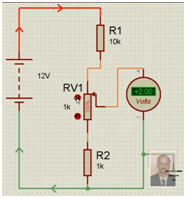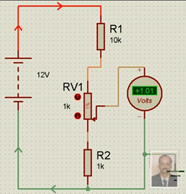• Capacitors: A capacitor is a linear passive component that is used to store an electrical charge. A capacitor generally provides reactance to the flow of current. A capacitor consists of a pair of electrodes between which there is an insulating dielectric material.

The stored charge is given by

Q = CV where C is the capacitive reactance and V is the applied voltage. Since current is the rate of flow of charge.             Therefore, the current through a capacitor is:

I = C dV/dt.

When a capacitor is connected in a DC circuit, or when a constant current flows through it, which is constant with time (zero frequency), the capacitor simply stores the whole charge and opposes the flow of current. Thus a capacitor blocks DC.

When a capacitor is connected in an AC circuit, or a time-varying signal flows through it (with non zero frequency), the capacitor initially stores the charge and later offers a resistance to the flow of charge. It can thus be used as a voltage limiter in the AC circuit. The resistance offered is proportional to the frequency of the signal.

### 2 Types of Capacitors

• Fixed Capacitors: They offer a fixed reactance to the flow of current. They can be the Mica capacitor which consists of mica as the insulating material. They can be nonpolarized ceramic capacitors which consist of ceramic plates coated with silver. They can be electrolyte capacitors that are polarized and used where a high value of capacitance is required.
• Variable Capacitors: They offer capacitance which can be varied by varying the distance between the plates. They can be air gap capacitors or vacuum capacitors.

Capacitance value can be either read directly on the capacitor or can be decoded using the given code. For ceramic capacitors, the 1st two letters denote the capacitance value. The third letter denotes the number of zeros and the unit is in Pico Farad and the letter denotes the tolerance value.

• Inductors:  An inductor is a passive electronic component that stores energy in the form of a magnetic field. It generally consists of a conductor coil, which offers a resistance to the applied voltage. It works on the basic principle of Faraday’s law of inductance, according to which a magnetic field is created when current flows through the wire and the electromotive force developed opposes the applied voltage. The stored energy is given by:

E = LI^2. Where L is the inductance measured in Henries and I is the current flowing through it.

It can be used as a choke to offer resistance to the applied voltage and store the energy or used in combination with a capacitor to form a tuned circuit, used for oscillations. In AC circuits, the voltage leads the current as imposed voltage takes some time to build up the current in the coil due to opposition.

### 2 Passive Non-Linear Components:

Diodes: A diode is a device that restricts current flow in only one direction. A diode is generally a combination of two differently doped regions forming a junction at the intersection such that the junction controls the flow of charge through the device.

6 Types of Diodes:

• PN Junction Diode: A simple PN junction diode consists of a p-type semiconductor mounted on an n-type semiconductor such that a junction is formed between the p and n types. It can be used as a rectifier that allows current flow in one direction through proper connection.
• Zener Diode: It is a diode made up of heavily doped p region compared to the n-region, such that it not only allows current flow in one direction but also allows current flow in the opposite direction, on the application of sufficient voltage. It is generally used as a voltage regulator.
• Tunnel Diode: It is a heavily doped PN junction diode where the current decreases with increasing forward voltage. The junction width is reduced with increasing impurity concentration. It is made from germanium or Gallium Arsenide.
• Light Emitting Diode: It is a special type of PN junction diode made from semiconductors like Gallium Arsenide, which emits light when a suitable voltage is applied. The light emitted by the LED is monochromatic, i.e. of a single color, corresponding to a particular frequency in the visible band of the electromagnetic spectrum.
• Photo Diode: It is a special type of PN junction diode whose resistance decreases when light falls on it. It consists of a PN junction diode placed inside a plastic.
• Switches: Switches are devices that allow the flow of current to the active devices. They are binary devices, which when completely on, allows the flow of current and when completely off, block the flow of current. It can be a simple toggle switch which can be a 2-contact or a 3 contact switch or a push-button switch.

### 2 Active Electronic Components:

Transistors: Transistors are devices which generally transform resistance from one part of the circuit to another. They can be voltage controlled or current controlled. A transistor can work as an amplifier or as a switch.

2 Types of Transistor:

• BJT or Bipolar Junction Transistor: A BJT is a current controlled device that consists of a layer of n-type semiconductor material sandwiched between two layers of p-type semiconductor material. It consists of three terminals – The emitter, base, and collector. The collector-base junction is less doped compared to the emitter-base junction. The emitter-base junction is forward biased whereas the collector-base junction is reverse biased in normal transistor operation.
• FET or Field Effect Transistor: A FET is a voltage-controlled device. The ohmic contacts are taken from the two sides of the n-type bar. It consists of three terminals – Gate, Drain, and Source.  The voltage applied across the Gate-Source and the Drain-Source terminal controls the flow of current through the device. It is generally a high resistance device. It can be JFET (junction Field effect transistor) which consists of an n-type substrate, on the side of which a bar of the opposite type is deposited or a MOSFET (Metal Oxide Semiconductor FET) which consists of an insulating layer of silicon oxide between the metallic Gate contact and the substrate.
• TRIACS or SCR: An SCR or Silicon Controlled Rectifier is a three-terminal device which is generally used as a switch in power electronics. It is a combination of two back to back diodes having 3 junctions. The current through the SCR flows because of the voltage applied across anode and cathode and is controlled by the voltage applied across the Gate terminal. It is also used as a rectifier in AC circuits.

So these are some of the important components in any electronic circuit. Apart from these active and passive components, there is one more component, which is of vital use in the circuit. That is the Integrated Circuit.

### What is an Integrated Circuit?

An Integrated Circuit is a chip or a microchip on which thousands of transistors, capacitors, resistors are fabricated. It can be an Amplifier IC, a timer IC, a waveform generator IC, a memory IC or a Microcontroller IC. It can be an analog IC with a continuous variable output or a Digital IC operating at a few defined layers. The fundamental building blocks of Digital ICs are the logic gates.

It can be available in different packages like Dual in Line Package(DIP) or Small Outline Package(SOP) etc.

### A Practical application of resistors – Potential Dividers

Potential dividers are frequently used in electronic circuits. Therefore it is desired that a thorough understanding of the same would greatly help in designing electronic circuits. Instead of deriving the voltages mathematically by applying Ohm’s law, the following example by assessing in ratio way, one would be able to quickly get the approximate voltage while attending to the R&D nature of work.

When two resistors of equal value (e.g. 6K both for R1 & R2) are connected across a supply, the same current will flow through them. If a meter is placed across the supply shown in the diagram it will register 12v concerning ground. If the meter is then placed between the ground (0v) and the middle of the two resistors it will read 6v. The battery voltage is then divided in half. Thus voltage across R2 for ground =6v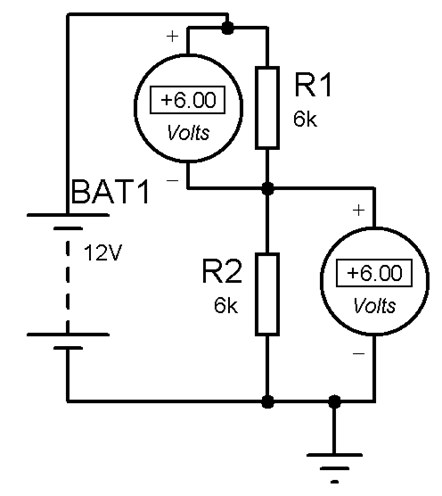Similarly

2. If the resistor values are changed to 4K(R1) and 8K (R2)the voltage at the center will be 8v for ground.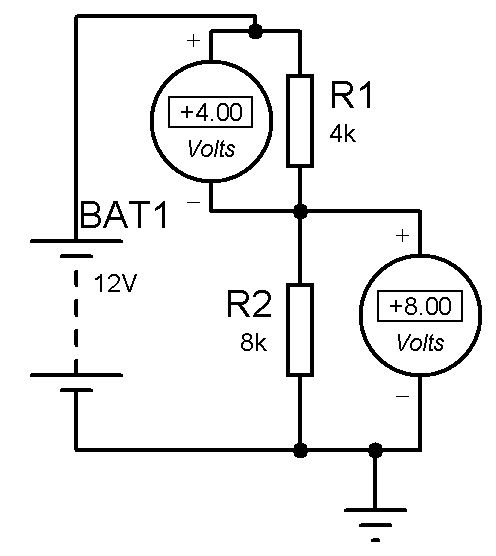3. If the resistor values are changed to 8K(R1) and 4K (R2) the voltage at the center will be 4v for ground.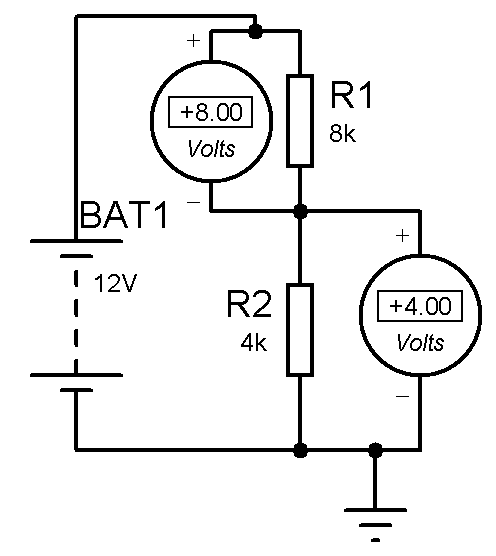The voltage at the center is better determined by the ratio of the two resistor values, though one can go by Ohms law to calculate to arrive at the same value.  Case-1 the ratio was 6K:6K = 1:1=6v:6v , Case-2 ratio 4k:8k= 1:2 =4v:8v and Case-3 ratio 8k:4k=  2:1=8v:4v

Conclusion:-In a potential divider, if the upper resistor value is lowered then the voltage at the center goes up (concerning ground). If the lower resistor value is lowered then the voltage at the center falls.

Mathematically but the voltage at the center can always be determined by the ratio of the two resistor values which is time-consuming and is given by the famous Ohms law formula V=IR

Let us see the example-2

V = { supply voltage /( R1+R2)}  X R2

V= {12v /(4K+8K)}R2

=(12/12000) x 8000

V = 8v

### Video on Testing of Electronic Components

Any further inputs are welcome to be added.

Photo Credit